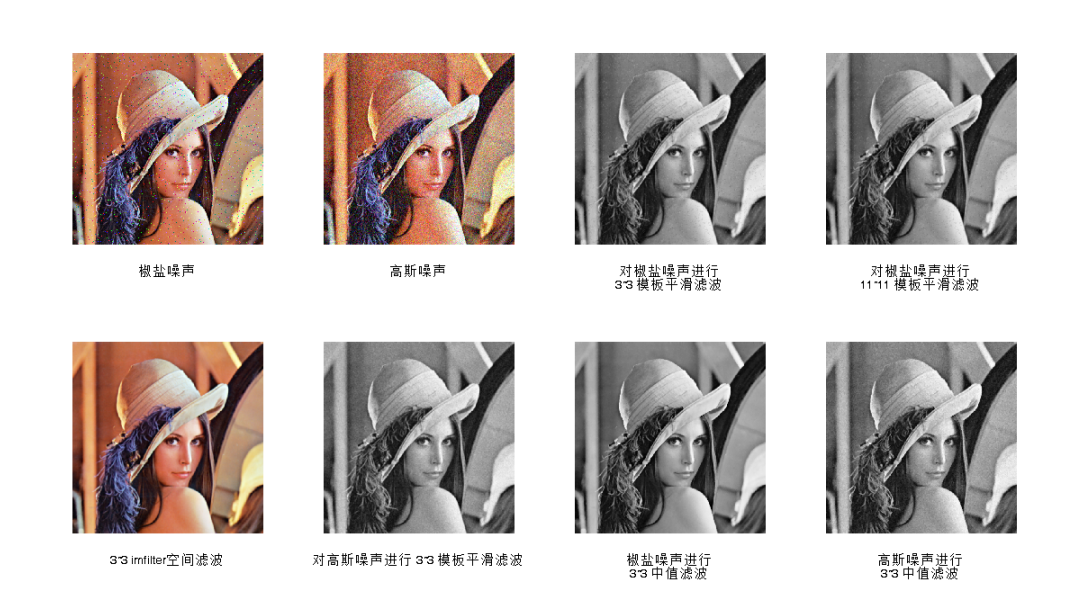• matlab自带滤波器函数小结(图像处理) 1 线性平滑滤波器 用MATLAB实现领域平均法抑制噪声程序： I=imread(' c4.jpg '); subplot(231) imshow(I) title('原始图像') flag = isrgb(I); if flag==true I=rgb2gray(I); ...

matlab自带滤波器函数小结(图像处理)   1 线性平滑滤波器 用MATLAB实现领域平均法抑制噪声程序： I=imread(' c4.jpg '); subplot(231) imshow(I) title('原始图像') flag = isrgb(I); if flag==true    I=rgb2gray(I); else     I=I; end I1=imnoise(I,'salt & pepper',0.02); subplot(232) imshow(I1) title(' 添加椒盐噪声的图像') k1=filter2(fspecial('average',3),I1)/255;          %进行3*3模板平滑滤波 k2=filter2(fspecial('average',5),I1)/255;          %进行5*5模板平滑滤波 k3=filter2(fspecial('average',7),I1)/255;          %进行7*7模板平滑滤波 k4=filter2(fspecial('average',9),I1)/255;          %进行9*9模板平滑滤波 subplot(233),imshow(k1);title('3*3 模板平滑滤波'); subplot(234),imshow(k2);title('5*5 模板平滑滤波'); subplot(235),imshow(k3);title('7*7 模板平滑滤波'); subplot(236),imshow(k4);title('9*9 模板平滑滤波'); 2 中值滤波器 用MATLAB实现中值滤波程序如下： I=imread(' c4.jpg '); flag = isrgb(I); if flag==true    I=rgb2gray(I); else     I=I; end J=imnoise(I,'salt&pepper',0.02); subplot(231),imshow(I);title('原图像'); subplot(232),imshow(J);title('添加椒盐噪声图像'); k1=medfilt2(J);            %进行3*3模板中值滤波 k2=medfilt2(J,[5,5]);      %进行5*5模板中值滤波 k3=medfilt2(J,[7,7]);      %进行7*7模板中值滤波 k4=medfilt2(J,[9,9]);      %进行9*9模板中值滤波 subplot(233),imshow(k1);title('3*3模板中值滤波'); subplot(234),imshow(k2);title('5*5模板中值滤波 '); subplot(235),imshow(k3);title('7*7模板中值滤波'); subplot(236),imshow(k4);title('9*9 模板中值滤波'); 3 状态统计滤波器：ordfilt2函数 Y=ordfilt2(X,order,domain) 由domain中非0元素指定邻域的排序集中的第order个元素代替X中的每个元素。Domain是一个仅包括0和1的矩阵，1仅定义滤波运算的邻域。 Y=ordfilt2(X,order,domain,S) S与domain一样大，用与domain的非0值相应的S的值作为附加补偿。 4 二维自适应除噪滤波器：wiener2函数 wiener2函数估计每个像素的局部均值与方差，该函数用法如下： J=wiener2(I,[M N],noise) 使用M×N大小邻域局部图像均值与偏差，采用像素式自适应滤波器对图像I进行滤波。 [J,noise]=wiener2(I,[M N]) 滤波前还有估计附加噪声的能量。 5 特定区域滤波 MATLAB图像处理工具箱中提供的roifilt2函数用于对特定区域进行滤波，其语法格式为： J=roifilt2(h,I,BW) 其功能是：使用滤波器h对图像I中用二值掩模BW选中的区域滤波。 J=roifilt2(I,BW,fun) J=roifilt2(I,BW,fun,P1,P2,…) 其功能是：对图像I中用二值掩模BW选中的区域作函数运算fun，其中fun是描述函数运算的字符串，参数为P1、P2、…。返回图像J在选中区域的像素为图像I经fun运算的结果，其余部分的像素值为I的原始值。 例：对指定区域进行锐化滤波的程序清单： I=imread('eight.tif'); c=[222 272 300 272 222 194]; r=[21 21 75 121 121 75]; BW=roipoly(I,c,r); h=fspecial('unsharp'); J=roifilt2(h,I,BW); subplot(1,2,1);imshow(I); subplot(1,2,2);imshow(J);

展开全文•双边滤波算法
• MATLAB常用的滤波函数比较：均值滤波和中值滤波 均值滤波中值滤波运行结果程序代码 均值滤波 均值滤波也称为线性滤波，其采用的主要方法为邻域平均法。是典型的线性滤波算法，它是指在图像上对目标像素给一个模板，...

均值滤波

均值滤波也称为线性滤波，其采用的主要方法为邻域平均法。是典型的线性滤波算法，它是指在图像上对目标像素给一个模板，该模板包括了其周围的临近像素（以目标像素为中心的周围8个像素，构成一个滤波模板，即去掉目标像素本身），再用模板中的全体像素的平均值来代替原来像素值。

中值滤波

中值滤波法是一种非线性平滑技术，它将每一像素点的灰度值设置为该点某邻域窗口内的所有像素点灰度值的中值. 中值滤波对脉冲噪声有良好的滤除作用，特别是在滤除噪声的同时，能够保护信号的边缘，使之不被模糊。这些优良特性是线性滤波方法所不具有的。此外，中值滤波的算法比较简单，也易于用硬件实现。

运行结果程序代码

J1=imnoise(I,'salt & pepper',0.02); %加均值为0，方差为0.02的椒盐噪声
J2=imnoise(I,'gaussian',0.02); %加均值为0，方差为0.02的高斯噪声。
g1=rgb2gray(J1);
g2=rgb2gray(J2);
figure('units','normalized','position',[0 0 1 1]);
subplot(2,4,1),imshow(J1),xlabel('椒盐噪声'); %显示有椒盐噪声图像
subplot(2,4,2),imshow(J2),xlabel('高斯噪声'); %显示有高斯噪声图像

% %  邻域平均法  neighborhood averaging

% imfilter 可进行多维图像（RGB等）进行空间滤波，且可选参数较多
% filter2 / medfilt2 只能对二维图像（灰度图）进行空间滤波,
% 两个函数结果类型不一样，只需要在I1=filter2(h,I)后面加上I1=uint8(I1)进行类型转换，结果就是一样的。

K1 = filter2(fspecial('average',3),g1); % 对椒盐噪声进行 3*3 模板平滑滤波
K2 = filter2(fspecial('average',11),g1);
k3 = imfilter(I,fspecial('average',3),'replicate');
K4 = filter2(fspecial('average',3),g2); % 对高斯噪声进行 3*3 模板平滑滤波

subplot(2,4,3),imshow(uint8(K1)),xlabel({'对椒盐噪声进行';'3*3 模板平滑滤波'});
subplot(2,4,4),imshow(uint8(K1)),xlabel({'对椒盐噪声进行';'11*11 模板平滑滤波'});
subplot(2,4,5),imshow(k3),xlabel('3*3 imfilter空间滤波');
subplot(2,4,6),imshow(uint8(K4)),xlabel('对高斯噪声进行 3*3 模板平滑滤波');

% 中值滤波
I1= medfilt2(g1,[3,3]); % 对有椒盐噪声图像进行5×5方形窗口中值滤波
I2= medfilt2(g2,[3,3]); % 对有高斯噪声图像进行5×5方形窗口中值滤波

subplot(2,4,7),imshow(I1),xlabel({'椒盐噪声进行';'3*3 中值滤波'});
subplot(2,4,8),imshow(I2),xlabel({'高斯噪声进行';'3*3 中值滤波'});

展开全文MATLAB 图像处理 滤波
• 双边滤波器的Matlab实现，附例程。简单的实现双边滤波器，是博客的附加例程 基本原理 保留边界细节，模糊变化不明显的区域 我们知道高斯滤波器可以起到模糊图像的作用，而上述的过程是选择性地进行模糊，在这里是对...
• matlab图像滤波代码全解，包括均值，中值，等等Matlab滤波
• 高斯滤波函数、双边滤波函数MATLAB实现高斯滤波函数双边滤波函数 自己编写滤波函数，剖析滤波内部结构 高斯滤波函数 clc; clear; close all; Image=imread('lena.bmp'); sigma1=0.6; sigma2=10; r=3; % 高斯模板...

高斯滤波函数、双边滤波函数的MATLAB实现

自己编写滤波函数，剖析滤波内部结构

高斯滤波函数

clc; clear; close all;

sigma1=0.6; sigma2=10; r=3;  % 高斯模板的参数
NoiseI= imnoise(Image,'gaussian'); % 加噪,添加高斯噪声
gausFilter1=fspecial('gaussian',[2*r+1 2*r+1],sigma1); % 高斯滤波器
gausFilter2=fspecial('gaussian',[2*r+1 2*r+1],sigma2);
result1=imfilter(NoiseI,gausFilter1,'conv'); % 使用卷积完成滤波
result2=imfilter(NoiseI,gausFilter2,'conv');
imshow(Image);title('原图');
figure;imshow(NoiseI);title('高斯噪声图像');
figure;imshow(result1);title('sigma1 =0.6高斯滤波');
figure;imshow(result2);title('sigma2 =10高斯滤波');
imwrite(uint8(NoiseI),'gr.bmp');
imwrite(uint8(result1),'gr1.bmp');
imwrite(uint8(result2),'gr2.bmp');
% 编写高斯滤波函数实现
[height,width]=size(NoiseI);
for x=-r:r
for y=-r:r
H(x+r+1,y+r+1)=1/(2*pi*sigma1^2).*exp((-x.^2-y.^2)/(2*sigma1^2));
end
end
H=H/sum(H(:));  %归一化高斯模板H
result3=zeros(height,width);            %滤波后图像
midimg=zeros(height+2*r,width+2*r);    %中间图像
midimg(r+1:height+r,r+1:width+r)=NoiseI;
for ai=r+1:height+r
for aj=r+1:width+r
temp_row=ai-r;
temp_col=aj-r;
temp=0;
for bi=1:2*r+1
for bj=1:2*r+1
temp= temp+(midimg(temp_row+bi-1,temp_col+bj-1)*H(bi,bj));
end
end
result3(temp_row,temp_col)=temp;
end
end
figure;imshow(uint8(result3));title('自己写的高斯滤波');
imwrite(uint8(result3),'gr3.bmp');

高斯噪声图像 ：Sigmal=0.6高斯滤波：Sigmal=10高斯滤波：自己写的高斯滤波：双边滤波函数

NoiseI= Image+0.05*randn(size(Image));  % 添加随机噪声
w=15; % 定义双边滤波窗口宽度
sigma_s=6; sigma_r=0.1; % 双边滤波的两个标准差参数
[X,Y] = meshgrid(-w:w,-w:w);
Gs = exp(-(X.^2+Y.^2)/(2*sigma_s^2)); % 计算邻域内的空间权值
[hm,wn] = size(NoiseI);
result=zeros(hm,wn);
for i=1:hm
for j=1:wn
temp=NoiseI(max(i-w,1):min(i+w,hm),max(j-w,1):min(j+w,wn));
Gr = exp(-(temp-NoiseI(i,j)).^2/(2*sigma_r^2)); % 计算灰度邻近权值
W = Gr.*Gs((max(i-w,1):min(i+w,hm))-i+w+1,(max(j-w,1):min(j+w,wn))-j+w+1); % W为空间权值Gs和灰度权值Gr的成绩
result(i,j)=sum(W(:).*temp(:))/sum(W(:));
end
end
subplot(1,3,1),imshow(Image),title('原始图像');
subplot(1,3,2),imshow(NoiseI),title('随机噪声图像');
subplot(1,3,3),imshow(result),title('双边滤波图像');
imwrite(NoiseI,'ng1.bmp');
imwrite(result,'ng2.bmp');

随机噪声图像：双边滤波图像：展开全文• 浅析matlab图像滤波

万次阅读 2017-03-09 13:24:23
浅析matlab图像滤波 1、首先我们要知道为什么要给图像滤波？  在尽量保留图像细节特征的条件下对目标图像的噪声进行抑制，是图像预处理中不可缺少的操作，其处理效果的好坏将直接影响到后续图像处理...

浅析matlab图像滤波

1、首先我们要知道为什么要给图像滤波？

在尽量保留图像细节特征的条件下对目标图像的噪声进行抑制，是图像预处理中不可缺少的操作，其处理效果的好坏将直接影响到后续图像处理和分析的有效性和可靠性

2、什么是图像滤波？

由于成像系统、传输介质和记录设备等的不完善，数字图像在其形成、传输记录过程中往往会受到多种噪声的污染。另外，在图像处理的某些环节当输入的像对象并不如预想时也会在结果图像中引入噪声。这些噪声在图像上常表现为一引起较强视觉效果的孤立像素点或像素块。一般，噪声信号与要研究的对象不相关它以无用的信息形式出现，扰乱图像的可观测信息。
对于数字图像信号，噪声表为或大或小的极值，这些极值通过加减作用于图像像素的真实灰度值上，对图像造成亮、暗点干扰，极大降低了图像质量，影响图像复原、分割、特征提取、图像识别等后继工作的进行。
要构造一种有效抑制噪声的滤波器必须考虑两个基本问题：能有效地去除目标和背景中的噪声;同时，能很好地保护图像目标的形状、大小及特定的几何和拓扑结构特征

3、常见的滤波器有哪些？

非线性滤波器：
一般说来，当信号频谱与噪声频谱混叠时或者当信号中含有非叠加性噪声时如由系统非线性引起的噪声或存在非高斯噪声等)，传统的线性滤波技术，如傅立变换，在滤除噪声的同时，总会以某种方式模糊图像细节(如边缘等)进而导致像线性特征的定位精度及特征的可抽取性降低。而非线性滤波器是基于对输入信号的一种非线性映射关系，常可以把某一特定的噪声近似地映射为零而保留信号的要特征，因而其在一定程度上能克服线性滤波器的不足之处。

线性滤波器：
也称均值滤波算法，主要思想为邻域平均法，即用几个像素灰度的平均值来代替每个像素的灰度。有效抑制加性噪声，但容易引起图像模糊，可以对其进行改进，主要避开对景物边缘的平滑处理。

中值滤波：
中值滤波由Turky在1971年提出，最初用于时间序列分析，后来被用于图像处理，并在去噪复原中取得了较好的效果。中值滤波器是基于次序统计完成信号恢复的一种典型的非线性滤波器，其基本原理是把图像或序列中心点位置的值用该域的中值替代，具有运算简单、速度快、除噪效果好等优点，曾被认为是非线性滤波的代表。然而，一方面中值滤波因不具有平均作用，在滤除诸如高斯噪声时会严重损失信号的高频信息，使图像的边缘等细节模糊；另一方面中值滤波的滤波效果常受到噪声强度以及滤波窗口的大小和形状等因素的制约，为了使中值滤波器具有更好的细节保护特性及适应性，人们提出了许多中值滤波器的改进算法。
标准中值滤波算法的基本思想是将滤波窗口内的最大值和最小值均视为噪声，用滤波窗口内的中值代替窗口中心像素点的灰度，在一定程度上抑制了噪声。实际上在一定邻域范围内具有最大或最小灰度值这一特性的，除了噪声点，还包括图像中的边缘点、线性特征点等。中值滤波以此作为图像滤波依据，其滤波结果不可避免地会破坏图像的线段、锐角等信息。因此，要找到一种既能实现有效滤除噪声，又能完整保留图像细节的滤波机制，仅考虑噪声的灰度特性是难以实现的。

Wiener维纳滤波：使原始图像和其恢复图像之间的均方误差最小的复原方法，是一种自适应滤波器，根据局部方差来调整滤波器效果。对于去除高斯噪声效果明显。

形态学滤波器：
随着数学各分支在理论和应用上的逐步深入，以数学形态学为代表的非线性滤波在保护图像边缘和细节方面取得了显著进展。形态学滤波器是近年来出现的一类重要的非线性滤波器，它由早期的二值形滤波器发展为后来的多值(灰度)形态滤波器，在形状识别、边缘检测、纹理分析、图像恢复和增强等领域了广泛的应用。
形态滤波方法充分利用形态学运算所具有的几何特征和良好的代数性质，主要采用态学开、闭运算进行滤波操作。从形态学基本原理可知，形态学的开运算会去掉图像上与结构元素的形态不相吻合的相对亮的分布结构，同时保留那些相吻合的部分;而闭运算则会填充那些图像上与结构元素不相吻合的相对暗的分布结构，同时保留那些相吻合的部分。因此他们都可以用来有效的提取特征和平滑像。值得注意地是，采用形态滤波器时，应根据不同的目的选择具有不同形状、大小和方向特性的结构元素。
此外，形态学开、闭运算都具有幂等性，这意味着一次滤波就己将所有特定于结构元素的噪声滤除千净，再次重复不会产生新的结果。这是一个经典方法(如线性卷积滤波、中值滤波)所不具备的性质。由于形态学运算是从图像的几何形态观点来进行图像处理的，因此这种优良的非线性滤波器能在滤波的同时，保持图像结构不被钝化。

4、图像去噪典的型算法及matlab实现

（1）、均值滤波matlab程序参考：

J=imnoise(I,'gaussian',0,0.005);%加入均值为0，方差为0.005的高斯噪声

subplot(2,3,1);imshow(I);

title('原始图像');

subplot(2,3,2); imshow(J);

title('加入高斯噪声之后的图像');

%采用MATLAB中的函数filter2对受噪声干扰的图像进行均值滤波

K1=filter2(fspecial('average',3),J)/255; %模板尺寸为3

K2=filter2(fspecial('average',5),J)/255;% 模板尺寸为5

K3=filter2(fspecial('average',7),J)/255; %模板尺寸为7

K4= filter2(fspecial('average',9),J)/255; %模板尺寸为9

subplot(2,3,3);imshow(K1);

title('改进后的图像1');

subplot(2,3,4); imshow(K2);

title('改进后的图像2');

subplot(2,3,5);imshow(K3);

title('改进后的图像3');

subplot(2,3,6);imshow(K4);

title('改进后的图像4');
（2）、二维自适应维纳滤波matlab程序参考：

J=imnoise(I,'gaussian',0,0.005); %加入均值为0，方差为0.005的高斯噪声

K2=wiener2(J,[3 3]); %对加噪图像进行二维自适应维纳滤波

K2=wiener2(J,[5 5]); %对加噪图像进行二维自适应维纳滤波

K2=wiener2(J,[7 7]); %对加噪图像进行二维自适应维纳滤波

K2=wiener2(J,[9 9]); %对加噪图像进行二维自适应维纳滤波

subplot(2,3,1);imshow(I);

title('原始图像');

subplot(2,3,2);imshow(J);

title('加噪图像');

subplot(2,3,3);imshow(K1);

title('恢复图像1');

subplot(2,3,4);imshow(K2);

title('恢复图像2');

subplot(2,3,5);imshow(K3);

title('恢复图像3');

subplot(2,3,6);imshow(K4);

title('恢复图像3');

（3）、matlab图像处理形态学滤波教程详细参考：
http://blog.csdn.net/zhangyibo123456789/article/details/60955182

：均值滤波、中值滤波以及二维自适应维纳滤波的详细比较（带图）
http://www.cnblogs.com/xiangshancuizhu/archive/2011/01/04/1925276.html

希望博友们能找到自己想要的滤波方式。

展开全文matlab图像处理 图像滤波
• 文件中包含test.m文件和两个函数以及一张测试图片。利用学习机会自己编写了均值滤波和中值滤波函数，并在test.m文件中运行，有详细代码注释，希望一起学习。
• matlab下输入命令doc 函数名就可以调出帮助文档，有详细的关于该函数的解释。以下是关于这3个滤波函数简要的总结。假定在图像处理中。matlab 图像处理 imfilter conv2 filter2
• MATLAB图像滤波去噪分析及其应用MATLAB图像滤波去噪分析及其应用第1章 图像颜色空间相互转换与MATLAB实现1．1 图像颜色空间原理1．1．1 RGB颜色空间1．1．2 YCbCr颜色空间1．1．3 YUV颜色空间1．1．4 YIQ颜色空间...
• Matlab中滤波操作的相关函数

万次阅读 2018-10-20 15:28:53
Matlab中与滤波相关的函数：imfilter 和fspecial。imfilter完成滤波操作，fspecial用于创建一些预定义的二维滤波器供imfilter调用。 imfilter函数 函数格式：g=imfilter(f, w, option1, option2,…) 参数说明： f是...Matlab 图像处理 空间域滤波
• 该楼层疑似违规已被系统折叠隐藏此楼查看此楼(1)均值滤波function imres = averageFilter(im,N) % imres为均值滤波后的矩阵，参数im为图像的路径，N为滤波模板if 3==size(im,3) %判断im是否为三维图像，若是，则转换...
• MATLAB 图像滤波去噪分析及其应用第1章　图像颜色空间相互转换与MATLAB实现1．1　图像颜色空间原理1．1．1　RGB颜色空间1．1．2　YCbCr颜色空间1．1．3　YUV颜色空间1．1．4　YIQ颜色空间1．1．5　HSV颜色空间...
• Matlab滤波函数

万次阅读 2015-01-10 11:28:10
MATLAB图像处理工具箱提供了基于卷积的图象滤波函数filter2，filter2的语法格式为： Y = filter2(h,X) 其中Y = filter2(h,X)返回图像X经滤波算子h滤波后的结果，默认返回图像Y与输入图像X大小相同。其实filter2和...
• MATLAB滤波函数的源代码

千次阅读 2017-05-24 13:52:51
目前比较经典的图像去噪算法主要有以下三种：   均值滤波算法：也称线性滤波，主要思想为邻域平均法，即用几个像素灰度 的平均值来代替每个像素的灰度。...%x是需要滤波图像,n是模板大小(即n×n)  function
• 1）噪声模拟：利用函数imnoise给图像‘eight.tif’分别添加高斯(gaussian)噪声和椒盐(salt & pepper)噪声。 2）空域滤波：对上述噪声图像进行不同模板大小的均值滤波和中值滤波，比较滤波效果。 3）最大值、最小值、...
• 用于数字图像处理的Matlab高斯滤波，此为单独封装的函数高斯滤波
• 压缩包里面有6个.m文件, blurring.m用于给图像增加抖动效果 inverseFilter.m是不考虑噪声的逆滤波 inverseFilterWithNoise.m是考虑噪声的逆滤波 wienerFiltering.m是维纳滤波 另外两个是辅助函数维纳滤波
• matlab对一幅图像进行算术滤波，几何滤波，谐波滤波，逆谐波滤波matlab
• MATLAB 自适应中值滤波函数adpmedian参考 冈萨雷斯 《数字图像处理》MATLAB版function f = adpmedian(g,Smax)if (Smax<=1)||(Smax/2==round(Smax/2))||(Smax~=round(Smax))error('Smax must be an odd integer &...
• 1、conv2函数 C=conv2(A,B,shape); %卷积滤波 参数说明： A：输入图像 B：卷积核 shape的可选值为full、same、valid。 1）当shape=full时，返回全部二维卷积结果，即返回B的大小为（ma+mb-1）x（na+nb-1）。 2）...matlab conv2 imfilter filter2
• 图像处理的基础函数，在此基础上可以完成双，多尺度滤波函数的编写，适合有一定图像处理基础的人员下载使用。图像处理 均值滤波
• MATLAB中值滤波图像处理，GUI界面加.m文件中值滤波图像
• Matlab图像滤波

千次阅读 2016-09-18 13:26:46
Matlab图像处理-滤波（高斯，中值，双边）的具体实现，涉及到了椒盐噪声的添加和去除。图像滤波 Matlab
•引导滤波代码...

matlab图像滤波函数matlab 订阅